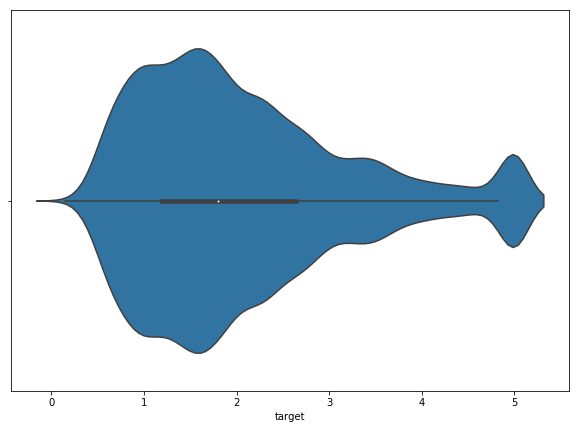Matplotlib and Seaborn are two of the most powerful and popular data visualization libraries in Python. Read on to learn how to create some of the most frequently used graphs and charts using Matplotlib and Seaborn.

(# Tip 5 )

8) In `.text` and `.annotate` methods there is a parameter `bbox` which takes a dictionary to set properties of box around the text. For `bbox`, you can get away with `pad`, `edgecolor`, `facecolor` and `alpha` for almost all cases.

9) In `.annotate` method there is a parameter for setting properties of an arrow, which you will be able to set if you have set `xytext` parameter, and it is `arrowprops`. It takes a dictionary as an argument, and you can get away with `arrowstyle` and`color`.

10) You can use use `matplotlib`'s `fill_between` or `fill_betweenx` to fill with a color between two curves. This can come in handy to highlight certain regions of a curve.

D] You should take your time thinking about how you should plot your data and which particular plot will get your message across the most.

```from matplotlib.pyplot import figure
figure(figsize=(10, 7))

plt.scatter('AveRooms', 'AveBedrms', data=data)
plt.plot(train_df['AveRooms'], Y, linewidth=1, color='red',
linestyle='-', alpha=0.8)

plt.xlabel("Avg Rooms  ->")
plt.ylabel("Avg BedRooms  ->")

plt.annotate("Possible outliers", xy=(144, 31), xytext=(160, 34),
arrowprops={'arrowstyle':'-[,widthB=4.0', 'color':
'black'},
'orange', 'alpha':0.4})

plt.annotate("Regression Line", xy=(80, 12), xytext=(120, 3),
arrowprops={'arrowstyle':'->', 'color': 'black',
'orange', 'alpha':0.4});
```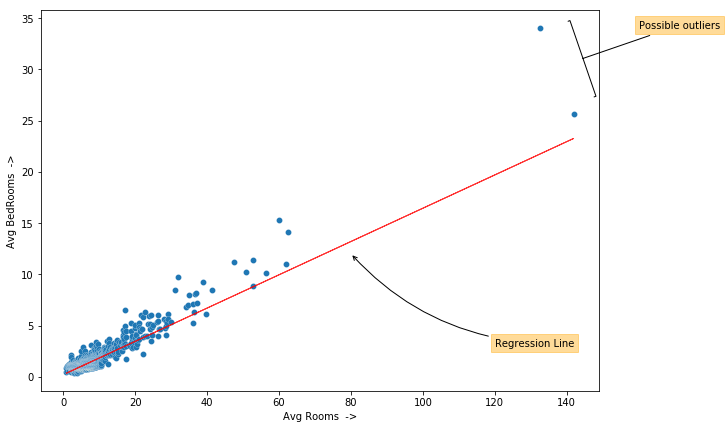Storytelling With Matplotlib (SWMat)

```swm = SWMat(plt)
plt.scatter(x, y, edgecolors='w', linewidths=0.3)
swm.line_plot(x, Y, highlight=0, highlight_color="#000088",
alpha=0.7, line_labels=["Regression Line"])
swm.title("'AveBedrms' and 'AveRooms' are highly correlated!",
ttype="title+")
swm.text("Taking both of them in regressioin process\nmight not be
necessary. We can either\n<prop color='blue'>take one of
them</prop> or <prop color='blue'>take average.</prop>",
position='out-mid-right', btw_line_dist=5)
swm.axis(labels=["Average Rooms", "Average Bedrooms"])```

# 'SWMat' has an `axis` method with which you can set some Axes
# properties such as 'labels', 'color', etc. directly.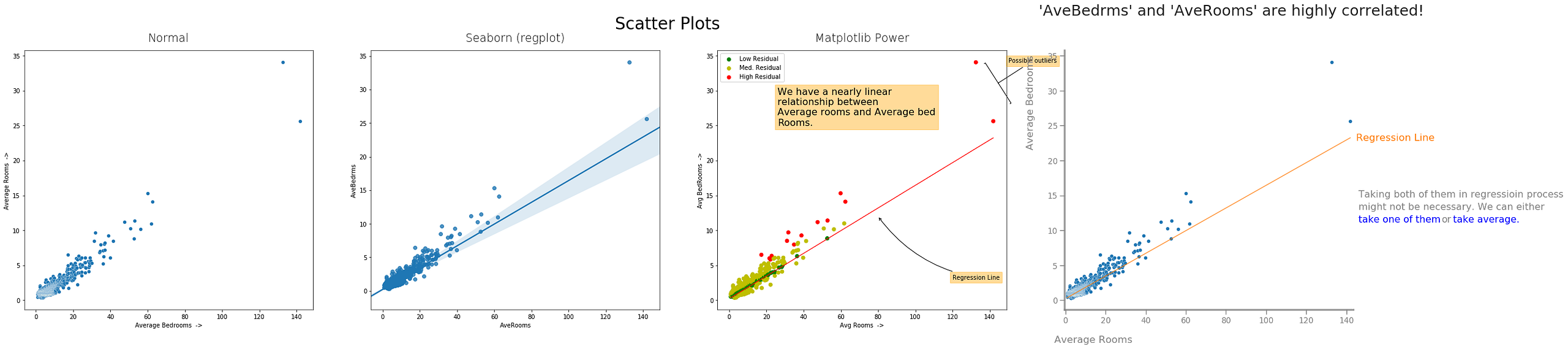1) Normal Matplotlib, 2) Seaborn, 3) Matplotlib Power, 4) Storytelling With Matplotlib

c) 2D-Histograms, Hex Plots and Contour Plots:

2D-Histograms and Hex Plots can be used to check relative density of data at particular position.
Contour plots can be used to plot 3D data in 2D, or plot 4D data in 3D. A contour line (or color strip in filled contour) tells us location where function has constant value. It makes us familiar with the whole landscape of variables used in plotting. For example it can be used in plotting cost function w.r.t. different theta’s in Deep Learning. But to make it you need a lot of data, to be accurate. As for plotting the whole landscape you will need data for all points in that landscape. And if you have a function for that landscape you can easily make these plots by calculating values manually.

```from matplotlib.pyplot import figure
figure(figsize=(10, 7))```

```plt.hist2d('MedInc', 'target', bins=40, data=train_df)
plt.xlabel('Median Income  ->')
plt.ylabel('Target  ->')
plt.suptitle("Median Income vs Target", fontsize=18);```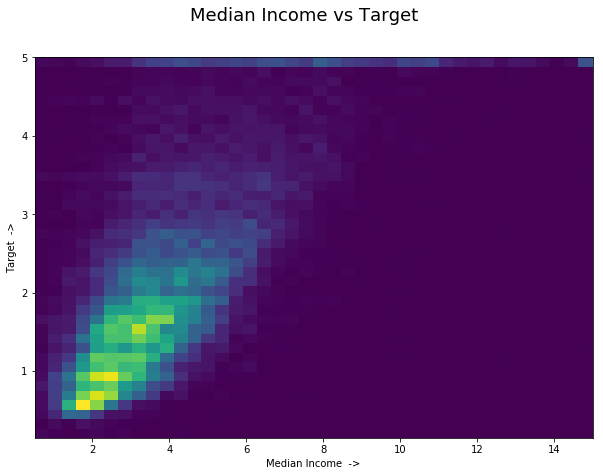But there is no separate Hex plot/2D-Hist plot method in `seaborn`, you can use `jointplot` method’s `kind` parameter for making a hex plot. For more info look into Joint Plots on `seaborn`.

(Tip #6)

11) A `colorbar` needs a `Mappable` object. Plots such as `Contour`, `Scatter` and `hist2d` gives them by default. There you can simply call `plt.colorbar()` and it will show a `colorbar` beside your plot. For other plots you can manually make a `colorbar` if you want to. [One example in ‘Hist’ section of Jupyter Notebook provided.]

E] Always try to choose a simple plot which can be easily understood by the masses.

```# Hexbin Plot:
from matplotlib.pyplot import figure
figure(figsize=(10, 7))

plt.hexbin('MedInc', 'target', data=train_df, alpha=1.0,
cmap="inferno_r")

plt.margins(0)
plt.colorbar()
plt.xlabel('Median Income  ->')
plt.ylabel('Target  ->')
plt.suptitle("Median Income vs Target", fontsize=18);```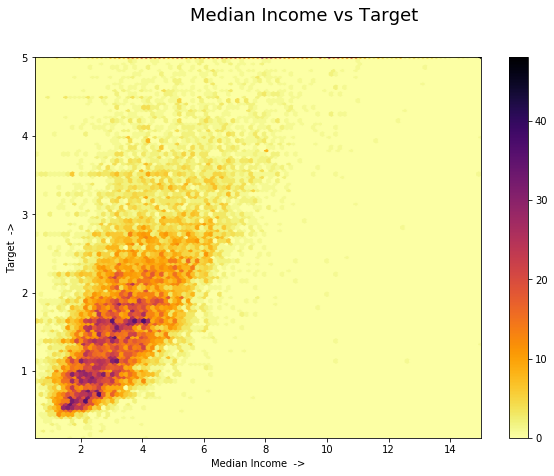```from matplotlib.pyplot import figure
figure(figsize=(10, 7))

plt.hist2d('MedInc', 'target', bins=40, data=train_df,
cmap='gist_heat_r')
plt.colorbar()
plt.xlabel('Median Income  ->')
plt.ylabel('Target  ->')
plt.suptitle("Median Income vs Target", fontsize=18)

plt.annotate("Most Blocks have low med.\nincome and lower target.",
xy=(5, 1.5), xytext=(10, 2),
arrowprops={'arrowstyle': '->', 'color': 'k'},
'edgecolor': 'orange'});```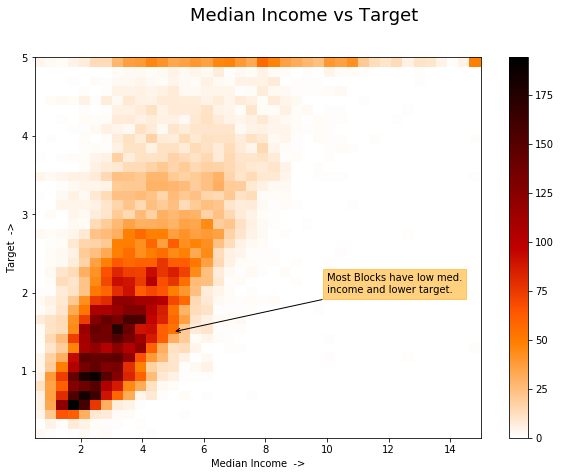Contour Plot: A contour plot is a way of visualizing 3D data on a 2D plot. In `matplotlib` there are two methods available, namely `.contour` and `.contourf`. The first one makes line contours and the second one makes filled contours. You can either pass an 2D matrix of z-values or pass in two 2D arrays X, Y for x-values and y-values and an 2D array for all corresponding z-values.

```# For contour plot
from matplotlib.pyplot import figure
figure(figsize=(10, 7))

plt.contourf(Z, levels=30, cmap="gist_heat_r")
plt.colorbar()

plt.suptitle("Target Contour", fontsize=16)
plt.title("(with Medium Income and Population)",
position=(0.6, 1.03))
plt.xlabel("Medium Income  ->")
plt.ylabel("Population  ->")```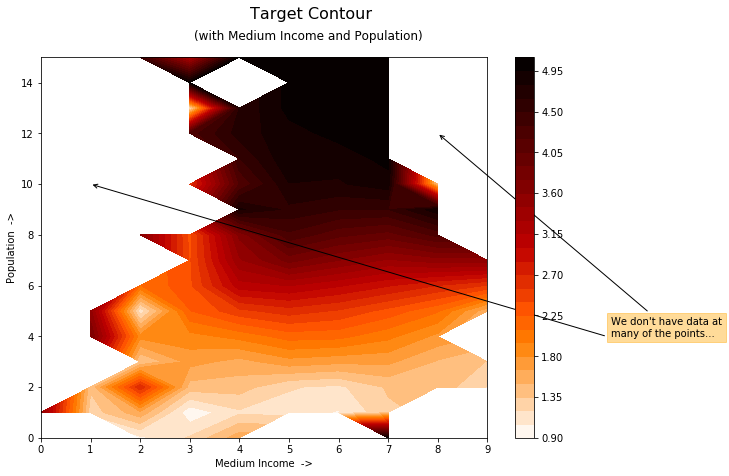d) Pair Plots:

`seaborn` provides a method `pairplot` with which you can plot all possible relational plots in one go. It can be used for quick view into relationship between all variables in your data, and also distribution of every variable.

`_ = sns.pairplot(train_df)`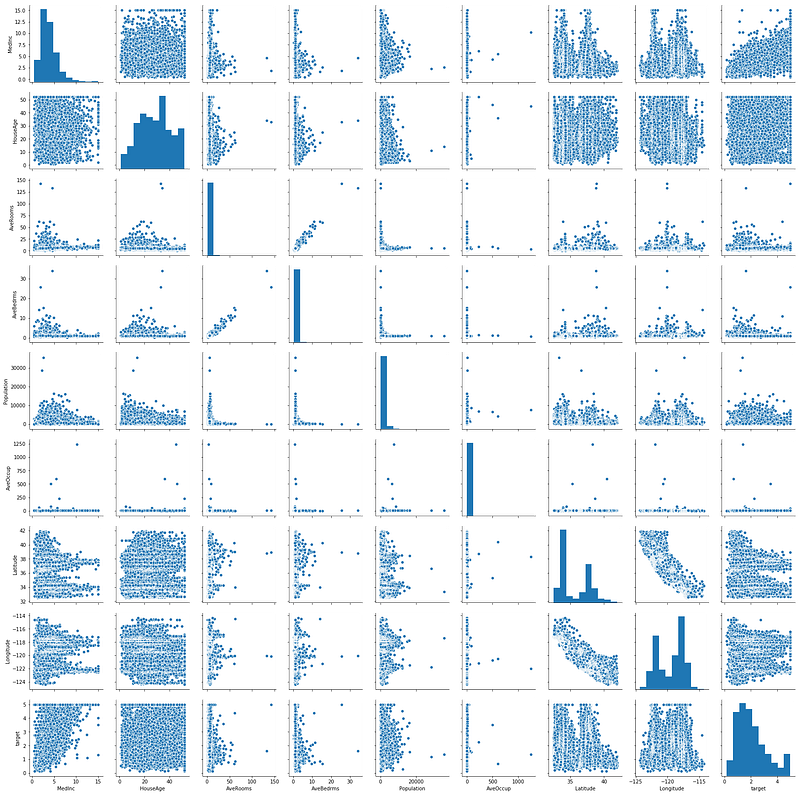### 4. Categorical PlotsCategorical plots are also necessary in Data Exploration step, as they tells us about how different classes of a variable are distributed in dataset. If we have sufficient data, we can make conclusions off these plots for different classes of that variable.

I have added Box Plot and Violin Plot here because of `seaborn`. In `seaborn` there are some parameters which you can use to use these methods with different categorical variables.

a) Bar Plot

Bar charts can be used to contrast between categories where their heights represent some value specific to that category.

```from matplotlib.pyplot import figure
figure(figsize=(10, 7))

plt.bar(np.sort(data.unique()), data.value_counts().sort_index(),
alpha=0.7) # You might need to sort; Be carefully with
# which values are being plotted with each
# other.

plt.xlabel("Target  ->")
plt.ylabel("Frequency  ->");

```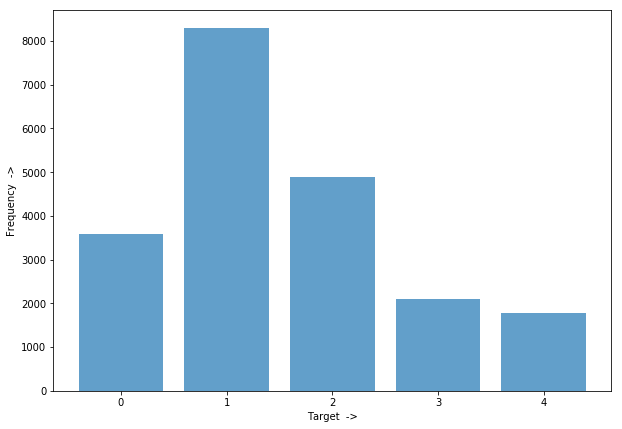(Tip #7)

12) If you have patch or object whose property you want to change, given in output of every `matplotlib` and `seaborn` functions, you can either change it by using `.set` function passing property name as string and property value to it, or you can directly use set function for that property like `set_color`, `set_lw`, etc.

F] There are nearly 8% men who are colorblind, nearly 1 in 10 and 0.5% of women. But still you should look out for them. `Orange-Blue` contrasts works for most of them.

```# Seaborn
from matplotlib.pyplot import figure
figure(figsize=(10, 7))

sns.barplot(np.sort(data.unique()),data.value_counts().sort_index())

plt.xlabel("Target  ->")
plt.ylabel("Frequency  ->");
```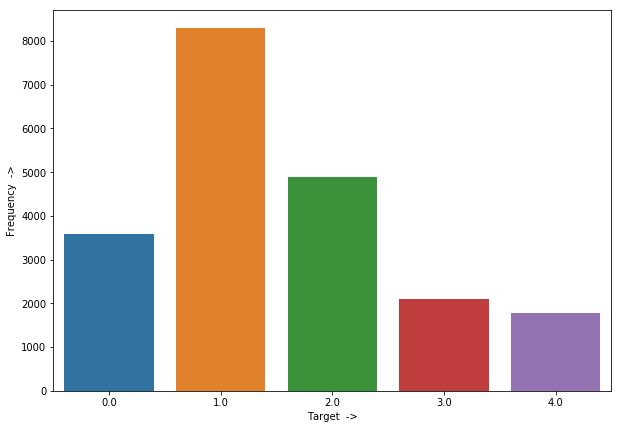```from matplotlib.pyplot import figure
figure(figsize=(10, 7))

plt.bar(np.sort(train_df['target_int'].unique()),
train_df['target_int'].value_counts().sort_index(),
alpha=0.7, width=0.6)

plt.grid(True, alpha=0.3)
plt.xlabel("Target  ->", fontsize=14)
plt.ylabel("Frequency  ->", fontsize=14)
plt.title("Target Frequencies", fontsize=18)

# Remove top and left spines:
ax = plt.gca() # Get current axis (gca)
ax.spines['right'].set_visible(False)
ax.spines['top'].set_visible(False)

counts = train_df['target_int'].value_counts().sort_index()
plt.annotate(str(counts), xy=(0, counts),
xytext=(0,counts+400), ha = 'center',
'orange', 'edgecolor': 'orange', 'alpha': 0.6},
arrowprops={'arrowstyle':"wedge,tail_width=0.5",
'alpha':0.6, 'color': 'orange'})
plt.annotate(str(counts), xy=(1, counts),
xytext=(1, counts+400), ha = 'center',
'orange', 'edgecolor': 'orange', 'alpha': 0.6},
arrowprops={'arrowstyle':"wedge,tail_width=0.5",
'alpha':0.6, 'color': 'orange'})
plt.annotate(str(counts), xy=(2, counts),
xytext=(2, counts+400), ha = 'center',
'orange', 'edgecolor': 'orange', 'alpha': 0.6},
arrowprops={'arrowstyle':"wedge,tail_width=0.5",
'alpha':0.6, 'color': 'orange'})
plt.annotate(str(counts), xy=(3, counts),
xytext=(3, counts+400), ha = 'center',
'orange', 'edgecolor': 'orange', 'alpha': 0.6},
arrowprops={'arrowstyle':"wedge,tail_width=0.5",
'alpha':0.6, 'color': 'orange'})
plt.annotate(str(counts), xy=(4, counts),
xytext=(4, counts+400), ha = 'center',
'orange', 'edgecolor': 'orange', 'alpha': 0.6},
arrowprops={'arrowstyle':"wedge,tail_width=0.5",
'alpha':0.6, 'color': 'orange'})
plt.xticks(ticks=[0, 1, 2, 3, 4], labels=["0 - 1", "1 - 2", "2 - 3",
"3 - 4", "4 - 5"], fontsize=12)
plt.ylim([0, 9500]);

```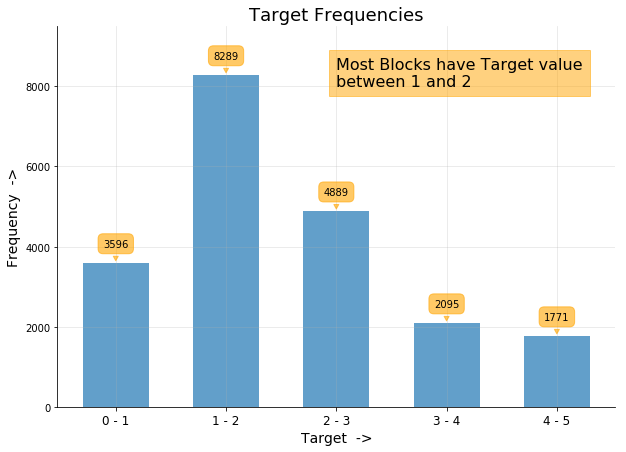Storytelling With Matplotlib (SWMat)

```swm = SWMat(plt)
swm.bar(cats, heights, highlight={"cat": [-1]}, highlight_type=
{"data_type": "incrementalDown"}, cat_labels=["0-1", "1-2",
"2-3", "3-4", "4-5"], highlight_color={"cat_color":
"#FF7700"}, annotate=True)
swm.axis(labels=["Target values", "Frequency"])
swm.title("About most expensive houses in California...")
swm.text("California is a sea-side state. As most\nexpensive houses
are at sea-side we\ncan easily predict these values if
we\nsomehow <prop color='blue'>combine 'Latitude'
and\n'Longitude' variables </prop>and separate sea\nside
houses from non-sea-side houses.",
btw_text_dist=.1);```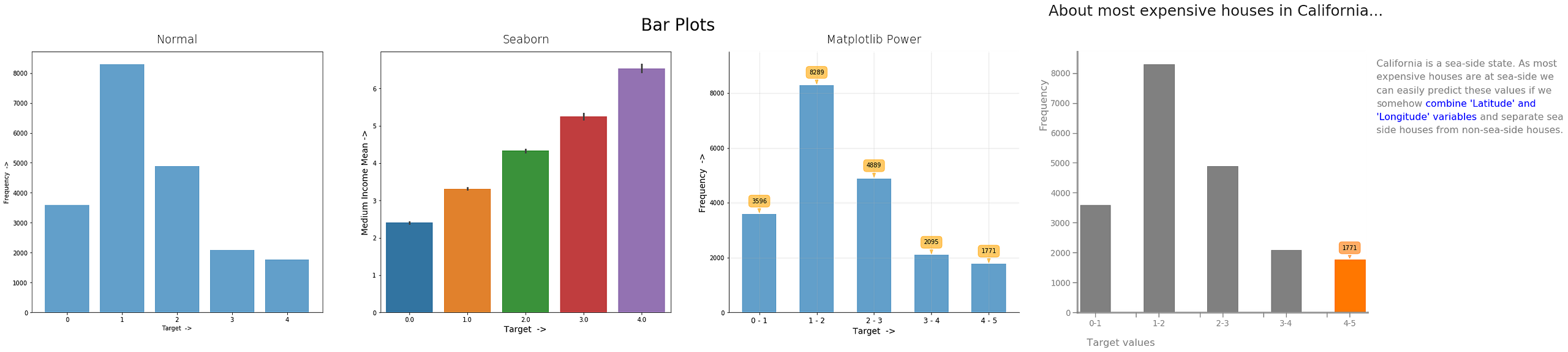1) Normal Matplotlib, 2) Seaborn, 3) Matplotlib Power, 4) Storytelling With Matplotlib

b) Box Plot

Box plot is a statistical version of distribution plot. It gives us range of different quartiles, mean, and extremas. Some possible use-case can be that with it you can identify variables in which you can find outliers if some points are way out of box-whisker’s range, or you can check for skew in distribution by relative placement of middle box in plot.

```from matplotlib.pyplot import figure
figure(figsize=(15, 7))

plt.boxplot(train_df['target'], vert=False)

plt.xlabel("<-  Target Values  ->")
plt.ylabel("Target");```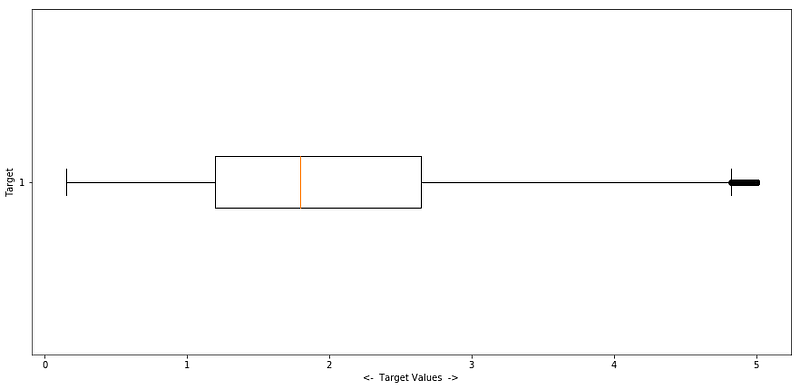```# With Seaborn:
from matplotlib.pyplot import figure
figure(figsize=(15, 7))

sns.boxplot(train_df['MedInc']);
```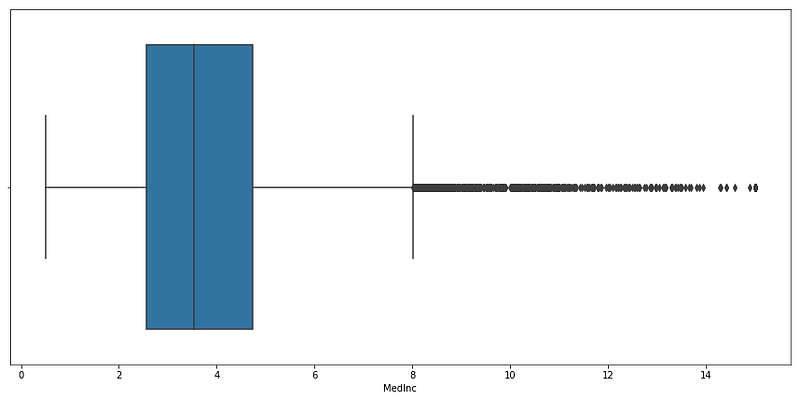(Tip #8 )

13) You can change x-limit, y-limit of your `Axes` by using functions `plt.xlim`, `plt.ylim`, `ax.set_xlim`, `ax.set_ylim`. You can also zoom in and out of your plot by using `plt.margings` or `ax.margins` as `plt.margins(x=2, y=-3)`.

14) You can use different styles for your plots from `plt.style.available` to give a different look to your plot, and activate them as `plt.style.use(stylename)`. Most used styles are `'fivethirtyeight'` and `ggplot`.

15) `seaborn` and `matplotlib` has many colormaps available which you can use to set color in plots for continuous variables. You can look for them here and here.

G] Highlight only the components of plot where you want your audience’s attention, and those parts only.

```from matplotlib.pyplot import figure
figure(figsize=(20, 7))

bp = plt.boxplot([x1, x2], vert=False, patch_artist=True,
flierprops={'alpha':0.6, 'markersize': 6,
'markeredgecolor': '#555555','marker': 'd',
'markerfacecolor': "#555555"},
capprops={'color': '#555555', 'linewidth': 2},
boxprops={'color': '#555555', 'linewidth': 2},
whiskerprops={'color': '#555555', 'linewidth': 2},
medianprops={'color': '#555555', 'linewidth': 2},
meanprops={'color': '#555555', 'linewidth': 2})```

plt.grid(True, alpha=0.6)
plt.title("Box Plots", fontsize=18)
plt.xlabel("Values ->", fontsize=14)
plt.ylabel("Features", fontsize=14)
plt.yticks(ticks=[1, 2], labels=['MedInc', 'Target'])

bp['boxes'].set(facecolor='#727FFF')
bp['boxes'].set(facecolor="#97FF67")

plt.text(11, 1.5, "There are many potential\nOutliers with respect
to\nMedian Income", fontsize=18,
bbox={'facecolor': 'orange', 'edgecolor': 'orange',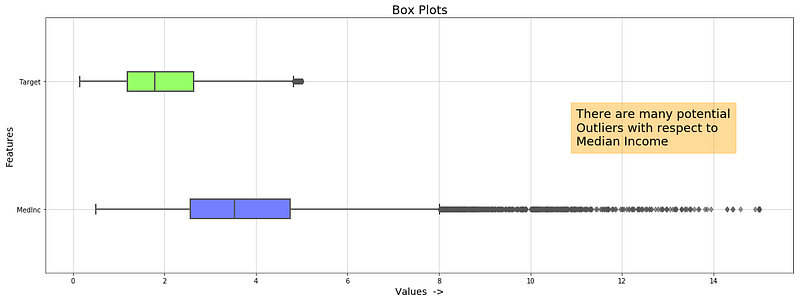Storytelling With Matplotlib (SWMat)

```swm = SWMat(plt)
bp = plt.boxplot([x1, x2], vert=False, patch_artist=True,
flierprops={'alpha':0.6, 'markersize': 6,
'markeredgecolor': '#555555','marker': 'd',
'markerfacecolor': "#555555"},
capprops={'color': '#555555', 'linewidth': 2},
boxprops={'color': '#555555', 'linewidth': 2},
whiskerprops={'color': '#555555', 'linewidth': 2},
medianprops={'color': '#555555', 'linewidth': 2},
meanprops={'color': '#555555', 'linewidth': 2})
plt.xlabel("Values  ->", fontsize=14)
plt.ylabel("Features", fontsize=14)
plt.yticks(ticks=[1, 2], labels=['MedInc', 'Target'])
bp['boxes'].set(facecolor='#727FFF')
bp['boxes'].set(facecolor="#97FF67");

swm.title("Many unusual outliers in 'MedInc' variable...")
swm.text(("It may be because of acquisition of sea side\n"
"places by very wealthy people. This <prop
color='blue'>aquisition\n"
"by many times greater earners</prop> and yet not much\n"
"number has made box plot like this."),btw_line_dist=.15,
btw_text_dist=.01)```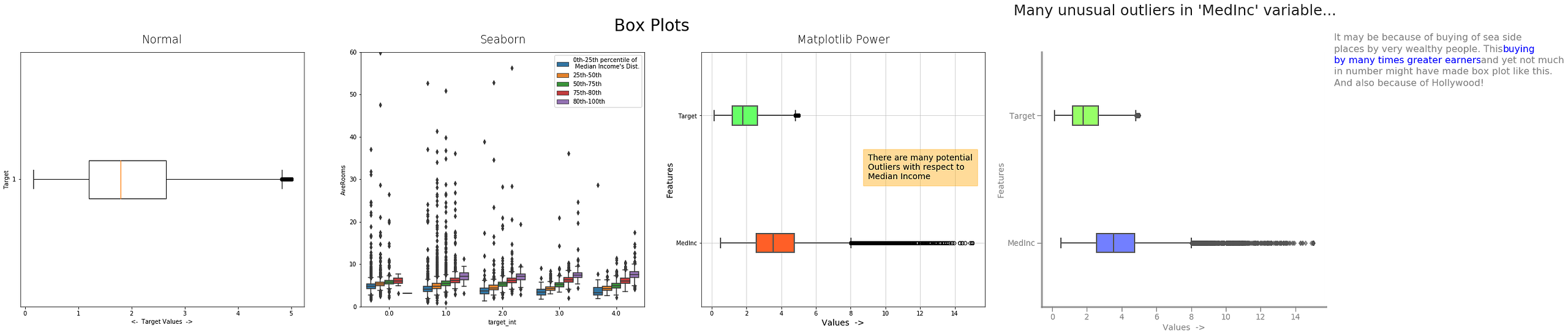1) Normal Matplotlib, 2) Seaborn, 3) Matplotlib Power, 4) Storytelling With Matplotlib

c) Violin Plot

Violin plot are extension of Box plot. It also has indicators of mean, extremas, and possibly different quartiles too. In addition to these it also shows probability distribution of the variable, on both sides.

```from matplotlib.pyplot import figure
figure(figsize=(10, 7))

plt.violinplot(train_df['target'])```

plt.title("Target Violin Plot")
plt.ylabel("Target values ->");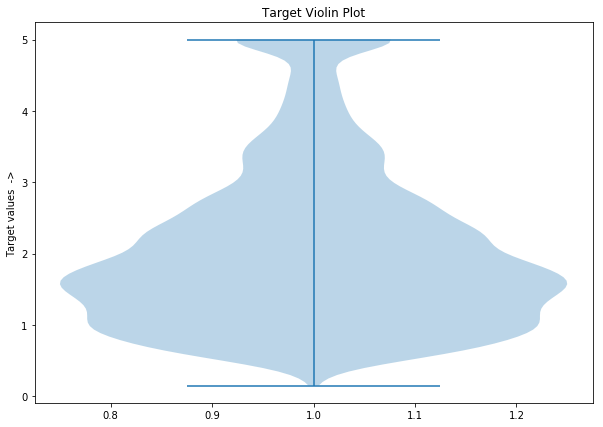```# With Seaborn
from matplotlib.pyplot import figure
figure(figsize=(10, 7))```

sns.violinplot(train_df['target']);